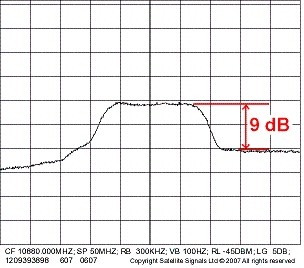VSAT information Latitude and longitude explanation Antenna beam-width calculator IET satellite communications summer school Views of the earth from various geo orbit positions Explanation and description of LNB Astra beam coverage O3b beams Link budget calculator Symbol rate Satellite beam design Noise temperature dB calculator

# EbNo measurement and EbNo Calculator

 Measured difference. (Co+No)/No e.g. 9.0 dB Result (1): calculated C/N   dB FEC code rate. e.g. 3/4 = 0.75 Bits per symbol. e.g. QPSK = 2 Result (2): calculated Eb/No   dB

The purpose of this page is to allow you to measure and then calculate the EbNo of a receive carrier using a spectrum analyser display.

The height of the carrier above the noise floor is (Co+No)/No dB     Note this is in units of dB.

The measured (C+N)/N is first converted into C/N. This is calculated result (1) above.

What FEC code rate is the carrier?    Example : Code rate 3/4 = 0.75

What is the number of transmission bits per symbol?  BPSK = 1,  QPSK = 2, 8-PSK =3, 16-QAM = 4, 32-APSK = 5Adjust your spectrum analyser so the display of the carrier looks something like this, preferably with the carrier occupying more of the height of the screen. In this case adjust the vertical scale to 2 dB per division instead of 5 dB per division as illustrated.

It is important that you can see the noise floor on either side of the carrier and that the top of the carrier is level. In the illustration the noise floor on the screen drops away at low frequencies. This is because the carrier is located at the bottom end of a transponder.

Before making any measurement pull out the cable from the analyser input and observe the noise floor.  It should go down by at least 20 dB.  If it goes down by a lesser amount your resulting Eb/No measurement will be in error.  You need to increase the input level to the analyser by measuring at a higher level point, to increase both the level of the noise and the level of the wanted carrier.  For example, remove any splitter and measure nearer to the LNA or LNB output.  Take care not to apply excessive power or DC power to the analyser input as you may destroy the analyser.  The analyser will probably have input attenuators.  Adjust these cautiously down to zero as appropriate.

Use a vertical scale interval that make the height of the carrier a significant proportion of the screen height to improve the accuracy of your EbNo measurement.

Use a resolution bandwidth that is significantly less than the carrier bandwidth. You can put video-averaging ON to clean up the short term variations.

Measure the height of the carrier relative to the noise floor.  Digital carriers look like noise and the noise floor is noise.  The top of the carrier is the carrier level plus the noise level. (Co+ No).     The noise floor is noise level (No).    The two readings you get will probably be in dBm.  This refers to the power in the current resolution bandwidth and is not accurate at all (in absolute terms) as spectrum analysers don't normally display noise measurements.   If your analyser is clever it may have a marker noise function.  If so, turn it on by selecting MARKER NOISE ON.   The results displayed will now show noise spectral density in dBm/Hz.  This is accurate and removes the bandwidth of the resolution bandwidth filter and also corrects for the analyser detector which is designed to measure the RMS values of CW (sinewave) carriers.  Ideally, you can use delta marker function so as to have a reference marker on top and put the delta marker on the noise floor nearby. The differential result will be (Co+No)/No in dB.

The Eb/No calculation is based on the carrier to noise spectral density ratio, the FEC code rate and the bits per symbol.

Feedback to me by e-mail Eric Johnston